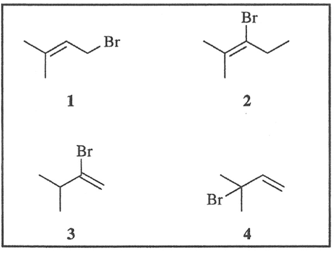# Problem: Which two compounds ionize with loss of bromide ion to form the same carbocation? a. 1 and 2 b. 2 and 3 c. 1 and 4 d. 3 and 4

###### FREE Expert Solution
90% (415 ratings)
###### Problem Details

Which two compounds ionize with loss of bromide ion to form the same carbocation?

a. 1 and 2

b. 2 and 3

c. 1 and 4

d. 3 and 4What scientific concept do you need to know in order to solve this problem?

Our tutors have indicated that to solve this problem you will need to apply the Carbocation Stability concept. You can view video lessons to learn Carbocation Stability. Or if you need more Carbocation Stability practice, you can also practice Carbocation Stability practice problems.

What is the difficulty of this problem?

Our tutors rated the difficulty ofWhich two compounds ionize with loss of bromide ion to form ...as high difficulty.

How long does this problem take to solve?

Our expert Organic tutor, Jonathan took 1 minute and 41 seconds to solve this problem. You can follow their steps in the video explanation above.

What professor is this problem relevant for?

Based on our data, we think this problem is relevant for Professor Nareddy's class at SCAR.